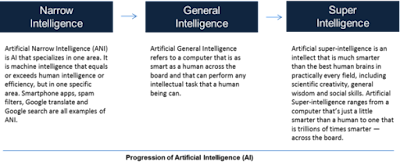Showing posts with label Floyd's Shortest Distance Algorithm. Show all posts
Showing posts with label Floyd's Shortest Distance Algorithm. Show all posts

### AI Glossary - Adjacency Matrix.

A binary relation over a finite set may be represented using an adjacency matrix.

If the cardinality of set A is n, the adjacency matrix for a relation on A is a nxn binary matrix, with a one for the I j-th element if the connection holds, and a zero otherwise.

The adjacency matrix is used by a variety of route and closure algorithms, either implicitly or explicitly.

If there are ones along the major diagonal, an adjacency matrix is reflexive, and symmetric if the I j-th element equals the j, i-th element for all I j pairings in the matrix.

The weighted adjacency matrix, which substitutes the zeros and ones with and costs, respectively, and utilizes this matrix to determine the shortest distance or minimal cost pathways between the components, is a generalization of this.

### Related Terms:

Floyd's Shortest Distance Algorithm, path matrix

~ Jai Krishna Ponnappan